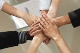# Remains constantly at 100%

ProgressAreaTop achievementsRank 1
Jordi asked on 28 Jul 2014, 10:06 PM
Good evening.

I've noticed that the progress bar does not work well when you step with decimal values ​​in Primary or Secondary Percent attribute.

The bar remains constantly at 100%

progress.SecondaryPercent = Math.Round (myPercent, 2);

progress.SecondaryPercent = Math.Round (myPercent, 0);

I think it's something that has to do with the locale. If working with the decimal encoding Spain (The decimal point is a comma) works bad, if I work with the american encoding works well.

Sorry for my poor English

Jordi

## 2 Answers, 1 is accepted

0Princy
Top achievementsRank 1
answered on 29 Jul 2014, 10:02 AM
Hi Jordi,

Please have a look into the sample code snippet which works fine at my end.

ASPX:
`<``asp:Button` `ID``=``"btnUpload"` `runat``=``"server"` `Text``=``"Upload"` `OnClick``=``"btnUpload_Click"` `/>`
`<``telerik:RadProgressManager` `ID``=``"Radprogressmanager1"` `runat``=``"server"` `/>`
`<``telerik:RadProgressArea` `ID``=``"RadProgressArea1"` `runat``=``"server"` `/>`

C#:
`protected` `void` `btnUpload_Click(``object` `sender, System.EventArgs e)`
`{`
`    ``int` `total = numbers.Count;`
`    ``decimal` `mypercent;`
`    ``RadProgressContext progress = RadProgressContext.Current;`
`    ``progress.Speed = ``"N/A"``;`
`    ``for` `(``int` `i = 0; i < total; i++)`
`    ``{`
`        ``progress.SecondaryTotal = total;`
`        ``progress.SecondaryValue = i;`
`        ``mypercent = (i * 100) / total;`
`        ``progress.SecondaryPercent = Math.Round(mypercent, 2);`
`        ``progress.CurrentOperationText = ``"Step "` `+ i.ToString();`
`        ``System.Threading.Thread.Sleep(30);`
`    ``}`
`}`

Thanks,
Princy.
0Jordi
Top achievementsRank 1
answered on 29 Jul 2014, 10:30 AM
Locale that you are using?

Try it with Spanish localization.

I think the progress bar is interpreting of 10,23 as 1023

If I do the test with American location if I work.

My message is not to ask for a solution (and I've found the solution by rounding the value.) was only an appreciation for I have lost a lot of time until I find the reason.

`progress.SecondaryTotal = datatable.Rows.Count;`

`foreach (DataRow row in datatable.Rows)`
`{`
`    ``NumReg++;`

`    ``double porcentage = ((double)NumReg / (double)datatable.Rows.Count) * 100;`
`    ``progress.SecondaryValue = NumReg;`
`    ``progress.SecondaryPercent = Math.Round(porcentage, 0);`

`    ``if (Response.IsClientConnected)`
`    ``{`
......
`        ``System.Threading.Thread.Sleep(100);`

`    ````} ....```

thank you very much
Tags
ProgressAreaJordi
Top achievementsRank 1Rank 1Rank 1